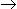# Chemical Engineering - Chemical Reaction Engineering

Exercise : Chemical Reaction Engineering - Section 4
46.
An imbalanced chemical reaction equation is against the law of
multiple proportion
conservation of mass
constant proportion
none of these
Explanation:
No answer description is available. Let's discuss.

47.
For the chemical reaction PQ, it is found that the rate of reaction doubles as the concentration of 'P' is doubled. If the reaction rate is proportional to Cpn, then what is the value of 'n' for this chemical reaction ?
1
2
3
0
Explanation:
No answer description is available. Let's discuss.

48.
Which of the following is a characteristic of an ideal plug flow reactor ?
Axial dispersion
Flat velocity profile
Uniform mixing
None of these
Explanation:
No answer description is available. Let's discuss.

49.
Velocity of a reaction depends upon the
nature of the reactants.
concentration of the reactants.
temperature at which the reaction is carried.
all (a), (b) and (c).